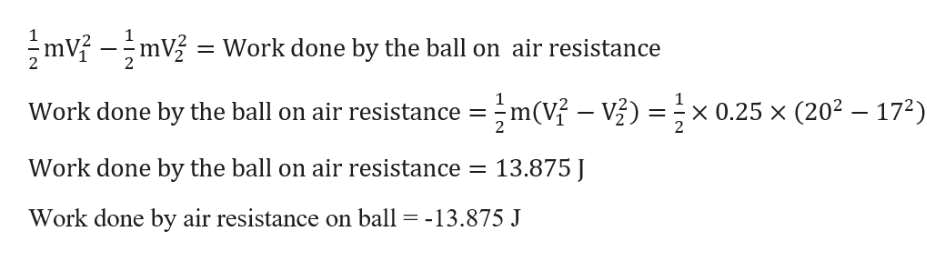# A boy throws a ball of mass 0.25 kg straight upwardwith an initial speed of 20 m/s When the ball returns to theboy, its speed is 17 m/s How much much work does airresistance do on the ball during its flight?

Question

A boy throws a ball of mass 0.25 kg straight upward
with an initial speed of 20 m/s When the ball returns to the
boy, its speed is 17 m/s How much much work does air
resistance do on the ball during its flight?

check_circle

Step 1

Given Information :

Initial velocity of the ball V1 = 20 m/s

Final Velocity of the ball V2 = 17 m/s

Mass of the ball m = 0.25 kg

When the ball moves against air resistance it will lose some of its energy in moving against air re...help_outlineImage TranscriptionclosemVmV Work done by the ball on air resistance m(V- V x 0.25 x (202 172) Work done by the ball on air resistance Work done by the ball on air resistance 13.875 Work done by air resistance on ball -13.875 J fullscreen

### Want to see the full answer?

See Solution

#### Want to see this answer and more?

Solutions are written by subject experts who are available 24/7. Questions are typically answered within 1 hour.*

See Solution
*Response times may vary by subject and question.
Tagged in

### Work,Power and Energy